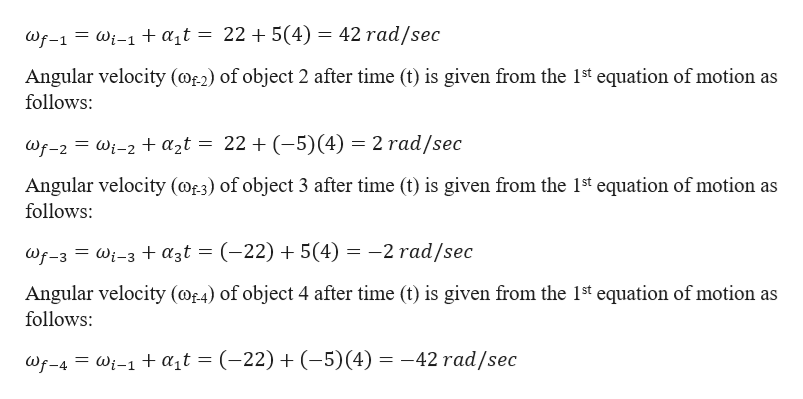# extbookour rotating objects at the same instant in time are listed in the table that fotime of 4 sInitial angular velocity weAngular acceleration a(a)(b)(c)(d)+22.0 rad/s+5.0 rad/s2-5,0 rad/s+5.0 rad/s2+22.0 rad/s-22.0 rad/s-22.0 rad/s-5.0 rad/shile you earn points based on the Point Potential Policy set by your instructor.Question Attempts: 1 of 6 used

Question
243 views

The inital angular velocity and the angular acceleration of four rotating objects at the same instant in time are listed in the table that follows. For each of the objects (a), (b), (c), and (d), determine the final angular speed after an elapsed time of 4 s.

check_circle

Step 1

Given information:

Initial angular velocity of object 1 (ωi-1) = +22 rad/s

Angular acceleration of object 1 (α1) = +5 rad/s2

Initial angular velocity of object 2 (ωi-2) = +22 rad/s

Angular acceleration of object 2 (α2) = -5 rad/s2

Initial angular velocity of object 3 (ωi-3) = -22 rad/s

Angular acceleration of object 3 (α3) = +5 rad/s2

Initial angular velocity of object 4 (ωi-4) = -22 rad/s

Angular acceleration of object 4 (α4) = -5 rad/s2

Angular velocity of each object after time (t) = 4 s

Step 2

Angular velocity (ωf-1) of object 1 after time (t) is given f...help_outlineImage Transcriptionclose= wi-1 at = 22 + 5(4) = 42 rad/sec Wf-1 Angular velocity (ae2) of object 2 after time (t) is given from the 1st equation of motion as follows: 2 rad/sec Wf-2 Wi-2 t azt = 22 + (-5) (4) Angular velocity (ae3) of object 3 after time (t) is given from the 1st equation of motion as follows: wf-3 Wi-3 + a3t = (-22) + 5(4) = -2 rad/sec Angular velocity (of4) of object 4 after time (t) is given from the 1st equation of motion as follows: = wi-1+ at =(-22) +(-5) (4) = -42 rad/sec Wf-4 fullscreen

### Want to see the full answer?

See Solution

#### Want to see this answer and more?

Solutions are written by subject experts who are available 24/7. Questions are typically answered within 1 hour.*

See Solution
*Response times may vary by subject and question.
Tagged in

### Angular Motion